# Test: Arrays, Stack, Queues & Linked List- 1

## 10 Questions MCQ Test Question Bank for GATE Computer Science Engineering | Test: Arrays, Stack, Queues & Linked List- 1

Description
Attempt Test: Arrays, Stack, Queues & Linked List- 1 | 10 questions in 30 minutes | Mock test for Computer Science Engineering (CSE) preparation | Free important questions MCQ to study Question Bank for GATE Computer Science Engineering for Computer Science Engineering (CSE) Exam | Download free PDF with solutions
QUESTION: 1

### Stack A has the entries a, b, c (with a on top). Stack B is empty. An entry popped out of stack A is pushed into stack B. An entry popped out of stack B can only be printed. In this arrangement, which of the following permutations of a, b, c are not possible to print? (i)   bac (ii)  bca (iii) cab (iv)  abc

Solution: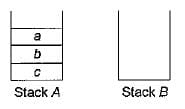a is popped from stack A and pushed to stack B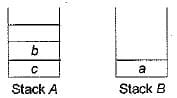b is popped from stack A and pushed to stack B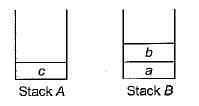c is also popped from stack A and pushed to stack B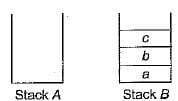c is popped from stack B and printed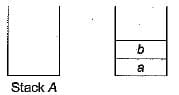Now, a can’t be popped out and printed since top of stack is ‘b’.

QUESTION: 2

### In the previous problem, if the stack A has 4 entries, then the number of possible permutations will be

Solution:

Total number of possible permutations for the previous problem is 5. For the four entries a, b, c, d the possibilities area, followed by permutations of a, b, c which is 5. b, followed by permutations of a, c, d which is 5. The other possibilities are c, b, a, d; c, d, b, a; c, b, d, a; d, c, b, a. Totally 14.

QUESTION: 3

### An item that is read as input can be either pushed to a stack and later popped and printed, or printed directly. Which of the following will be the output if the input is the sequence of items -1, 2, 3, 4, 5?

Solution:

The item can be pushed to stack and later popped and printed, or printed directly. 1, 2, 3, 4, 5 is the input then (a) is not possible because once pushed 1 is printed after 2.
(c) and (d) are not possible.
Hence, (b) is the output.

QUESTION: 4

The concatenation of two lists is to be performed in O(1) time. Which of the following implementations of a list could be used?

Solution:

In circular doubly linked list, one does not need to traverse the whole list to find the end of the list.
The second list can be concatenated at any location. Only fix number of pointers need to be changed. Hence can be done in O(1) time.

QUESTION: 5

Which of the following is essential for converting an infix expression to the postfix form efficiently?

Solution:

Operator stack is used to convert infix expression to postfix form whereas operand stack is used to convert postfix to infix notation.

QUESTION: 6

What can we say about the array representation of a circular queue when it contains only one element?

Solution:

If a queue contains an element Front = Rear can neither be NULL nor be -1.
So, option (a) and (c) are wrong.
Since, it is a circular queue and bottleneck case arises in case of two elements where Front = Rear + 1 and Front = Rear - 1 both are possible.
So, what all we can say is Front = Rear ≠ NULL if only one element is there in circular queue.

QUESTION: 7

In a compact single dimensional array representation for lower triangular matrices (i.e. all the elements above the diagonal are zero) of size n x n, non-zero elements (i.e. elements of the lower triangle) of each row are stored one after another, starting from the first row, the index of the (i, j)th element of the lower triangular matrix in this new representation is

Solution:

LOC (i, j) formula of lower triangular matrix used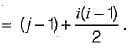QUESTION: 8

Let A be a two-dimensional array declared as follows:
A : array [1 ... 10] [1... 15] of integer;
Assuming that each integer takes one memory location. The array is stored in row-major order and the first element of the array is stored at location 100, what is the address of the element A [i] [j] ?

Solution:

The array is stored in row-major order, that means the elements are stored in the memory row-wise.
Suppose we have the 2D array
1 2 3
4 5 6
It will be stored in the memory as 1 2 3 4 5 6
If array is stored as column-major order then it will be stored as 1 4 2 5 3 6

Let A be a 2D array, A: array [b1 …. u1] [b2 …… u2], then
To find the location of A[i][j] for a 2D array stored in Row-major order use the following formula:

Loc A[i][j] = L + [ (i - b1) (u2 - b2 +1) + (j  - b2) ] x M

L - base address of the array, i.e. address of the first element of the array

M - Memory Size, here each integer takes only 1 memory location therefore M = 1

Loc A[i][j] = 100 + [ (i - 1) (15 - 1 +1) + (j  - 1) ] x 1

= 100 + (i - 1) 15 + j -1 = 15i + j + 84

QUESTION: 9

An n x n array v is defined as follows: v[i, j] = i - j for all i, j, 1 < i < n, 1 < j < n.The sum of the elements of the array v is:

Solution:

Array is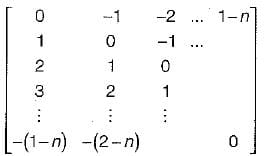Sum = 0 + 1 + 2 + ... + (-1) + (-2) + ... = 0.

QUESTION: 10

Suppose you are given an array s[1...n] and a procedure reverse (s, i, j) which reverses the order of elements in between positions i and j (both inclusive). What does the following sequence do, where 1 < k < n:
reverse (s, 1, k):
reverse (s, k + 1, n):
reverse (s, 1, n);

Solution:

Effect of the given 3 reversals for any k is equivalent to left rotation of the array of size n by k.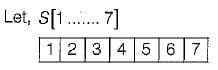∴ n = 7, k = 2
reverse (S, 1, 2) we get [2, 1, 3, 4, 5, 6, 7]
reverse (S, 3, 7) we get [2, 1, 7, 6, 5, 4, 3]
reverse (S, 1, 7) we get [3, 4, 5, 6, 7, 1,2]Use Code STAYHOME200 and get INR 200 additional OFF Use Coupon Code